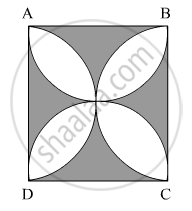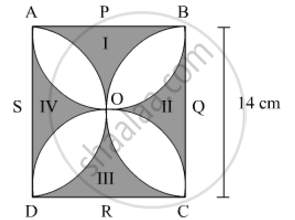Share

# In Fig. 4, ABCD is a square of side 14 cm. Semi-circles are drawn with each side of square as diameter. Find the area of the shaded region. - CBSE Class 10 - Mathematics

ConceptProblems Based on Areas and Perimeter Or Circumference of Circle, Sector and Segment of a Circle

#### Question

In Fig. 4, ABCD is a square of side 14 cm. Semi-circles are drawn with each side of square as diameter. Find the area of the shaded region.

(use pi=22/7)#### SolutionLet the four shaded regions be I, II, III and IV and the centres of the semicircles be P, Q, R and S, as shown in the figure.

It is given that the side of the square is 14 cm.

Now,

Area of region I + Area of region III = Area of the square − Areas of the semicircles with centres S and Q

=14×142×1/2×π×72          ( Radius of the semicircle=7 cm)

=192-49xx22/7

=196154

=42 cm2

Similarly,

Area of region II + Area of region IV = Area of the square − Areas of the semicircles with centres P and R.

=14×142×1/2×π×72    ( Radius of the semicircle=7 cm)

=19649×22/7

=196154

=42 cm2

Thus,
Area of the shaded region = Area of region I + Area of region III + Area of region II + Area of region IV

= 42 cm2 + 42 cm2

= 84 cm2

Is there an error in this question or solution?

#### Video TutorialsVIEW ALL 

Solution In Fig. 4, ABCD is a square of side 14 cm. Semi-circles are drawn with each side of square as diameter. Find the area of the shaded region. Concept: Problems Based on Areas and Perimeter Or Circumference of Circle, Sector and Segment of a Circle.
S